Published: 30 June 2017

# Attribute recognition model and its application of risk assessment for slope stability at tunnel portal

Qian Zhang1
Jing-chun Wang2
Hai-xia Zhang3
1Structure Health Monitoring and Control Institute, Shijiazhuang Tiedao University, Shijiazhuang, 050043, China
2Shijiazhuang Tiedao University, Shijiazhuang, 050043, China
3Beijing General Municipal Engineering Design and Research Institute Co. Ltd., 100082 Beijing, China
Corresponding Author:
Jing-chun Wang
Views 190

#### Abstract

Due to large span and complex construction technology, the portal section of tunnel is vulnerable to the threats of latent slope failures such as landslide, spalling and collapse. In the present paper, to ensure the construction safety of tunnel, the attribute recognition model of risk assessment for the slope stability at tunnel portal was established by using attribute mathematics theory. The integrated degree of rock mass, lithological feature, geological structural feature, weathering degree, seismic intensity, gradient, rainfall and construction factors were selected as the indices of the risk assessment for the stability slope at tunnel portal with grading criteria of each index. Moreover, the weights of the assessment indices were determined by the judgment matrix constructed from analytic hierarchy process. The attribute measurement functions were used to compute attribute measurement of single index and synthetic attribute measurement. The identification and classification of risk assessment for the slope stability at tunnel portal were assessed using the confidence criterion. For practical purposes, the stability of slope located in portal of an actual Tunnel, i.e., Jiefangcun Tunnel in Cheng-Lan Railway, was evaluated based on the previously established attribute recognition model. The corresponding risk treatment measures were then proposed based on the assessment results and monitor results to ensure the construction safety of tunnel portal.

## 1. Introduction

Due to large span and complex construction technology, the portal section of tunnel is vulnerable to the threats of latent slope failures such as landslide, spalling and collapse. In addition, the surrounding rock of tunnel portal has shallow burial depth, poor stability and complex geological structural conditions. Some studies conducted with the behavior of slope stability at tunnel portal were due to Barisone , Giani , Quek and  Lee  and Peila . These studies were very helpful in understanding the behavior of slope stability at tunnel portal. As to the analysis of the risk assessment on engineering, “Guidelines for Tunnel Risk Management” was proposed by the International Tunneling Association . Many studies were subsequently carried out to analyze the risk of seismic damage of tunnel portal, stability of slope and water inrush by using fuzzy analytical method and extension assessment method [7-16].

However, few studies are concerned on the most direct factor related to slope safety, and very limited data are available for the assessment systems reflecting the change of slope condition. Due to the random determination of membership function to a certain extent, the conditions of unclear classification and unreasonable results may be analyzed by using fuzzy analytical method . Based on the concepts of attribute set, attribute measure space and ordered partition class, Cheng  proposed the attribute recognition model to solve the comprehensive assessment. Recently, the attribute mathematics theory was applied successfully in the field of rock engineering. However, few relevant studies on slope stability at tunnel portal derived from attribute mathematics theory were conducted. Therefore, to evaluate the slope stability at tunnel portal, there is a need to establish the attribute recognition model of risk assessment for the slope stability at tunnel portal by using attribute mathematics theory.

In the present paper, the integrated degree of rock mass, lithological feature, geological structural feature, weathering degree, seismic intensity, gradient, rainfall and construction factors were selected as the indices of the risk assessment for stability slope at tunnel portal with grading criteria of each index. Moreover, the weights of the assessment indices were determined by using the judgment matrix. The attribute measurement functions were used to compute the attribute measurement of single index and synthetic attribute measurement. The identification and classification of risk assessment for the slope stability at tunnel portal were assessed by using the confidence criterion. For practical purposes, the stability of slope located in portal of an actual Tunnel, i.e., Jiefangcun Tunnel in Cheng-Lan Railway, was evaluated based on the previously established attribute recognition model. Based on the assessment results and monitor results, the corresponding risk treatment measures were then proposed to ensure the construction safety of tunnel portal.

## 2. Attribute recognition model of risk assessment for slope stability at tunnel portal

In practice, attribute mathematics theory is commonly used to analyze the risk of slope stability at tunnel portal. Following the suggestion of Cheng , the attribute comprehensive assessment system can be divided into three subsystems: attribute measure system of single index, comprehensive attribute measure system of multiple indices and attribute recognition analysis.

The risk assessment for slope stability at tunnel portal is a comprehensive assessment system with discrimination for slope instability by using the influencing factors. $X$ is assumed to be the evaluated object space, and the evaluated object ${x}_{i}$($i=$1, 2,…, $n$) has $m$ evaluated indices ${I}_{j}$ ($j=$1, 2,…, $m$). The value of the $j$-th evaluated index ${I}_{j}$ in ${x}_{i}$, ${t}_{j}$, is divided into $K$ kinds of assessment grades ${C}_{k}$($k=$1, 2,…, $K$). The attribute space $F$ adopted as {grades of slope stability at tunnel portal} is assumed to equal to $\left\{{C}_{1},{C}_{2},\dots ,{C}_{K}\right\}$, where each kind of circumstance is the attribute set.

The corresponding attribute measures of different attribute sets satisfying additivity rule can be obtained from the attribute arithmetic. The single-index attribute measure ${\mu }_{xjk}$ shows that the measured value of the $j$-th evaluated index ${I}_{j}$ of evaluated object, ${t}_{j}$, can be computed with the size of attribute ${C}_{k}$, whereas the comprehensive attribute measure ${\mu }_{xk}$ shows that the evaluated object can be calculated using the size of grade ${C}_{k}$ .

### 2.1. Analysis of single-index attribute measure

Based on the determination method of attribute measure ${\mu }_{xjk}=\mu$ (${x}_{ij}\in {C}_{k}$) ($1\le k\le K$), the attribute measure functions (see Table 1) can be established.

Table 1Grade subdivision of single index

 Indices Assessment grades ${C}_{1}$ ${C}_{2}$ … ${C}_{K}$ ${I}_{1}$ ${a}_{10}$-${a}_{11}$ ${a}_{11}$-${a}_{12}$ … ${a}_{1K-1}$-${a}_{1K}$ or >(<) ${a}_{1K}$ ${I}_{2}$ ${a}_{20}$-${a}_{21}$ ${a}_{21}$-${a}_{22}$ … ${a}_{2K-1}$-${a}_{2K}$ or >(<) ${a}_{2K}$ … … ${I}_{m}$ ${a}_{m0}$-${a}_{m1}$ ${a}_{m1}$-${a}_{m2}$ … ${a}_{mK-1}$-${a}_{mK}$ or >(<) ${a}_{mK}$

In Table 1, ${a}_{jk}$ ($k=$0, 1,…, $K$) is the upper or limiting value in the range interval of assessment gradessatisfying ${a}_{j0}<{a}_{j1}<\dots <{a}_{jK}$ or ${a}_{j0}>{a}_{j1}>\dots >{a}_{jK}$. One obtains:

1
${b}_{jk}=\frac{{a}_{jk-1}+{a}_{jk}}{2},$
2
${d}_{jk}=\mathrm{m}\mathrm{i}\mathrm{n}\left\{\left|{b}_{jk}-{a}_{jk}\right|,\left|{b}_{jk+1}-{a}_{jk}\right|\right\},$

where the value of $k$ presented in Eq. (1) is adopted as $k=$1, 2,…, $K$ and the value of $k$ presented in Eq. (2) is taken as $k=$1, 2, …, $K-1$.

For the case of ${a}_{j0}>{a}_{j1}>\dots >{a}_{jK}$, the attribute measure functions of single index ${\mu }_{xjk}\left(t\right)$ can be determined in the following forms:

3
${\mu }_{xj1}\left(t\right)=\left\{\begin{array}{l}0,t<{a}_{j1}-{d}_{j1},\\ \frac{t-{a}_{j1}+{d}_{j1}}{2{d}_{j1}},{a}_{j1}-{d}_{j1}\le t\le {a}_{j1}+{d}_{j1},\\ 1,t>{a}_{j1}+{d}_{j1},\end{array}\right\$
4
${\mu }_{xjk}\left(t\right)=\left\{\begin{array}{l}0,t<{a}_{jk}-{d}_{jk},\\ \frac{t-{a}_{jk}+{d}_{jk}}{2{d}_{jk}},{a}_{jk}-{d}_{jk}\le t\le {a}_{jk}+{d}_{jk},\\ 1,{a}_{jk}+{d}_{jk}{a}_{jk-1}+{d}_{jk-1},\end{array}\right\$
5
${\mu }_{xjK}\left(t\right)=\left\{\begin{array}{l}1,t<{a}_{jK-1}-{d}_{jK-1},\\ \frac{{a}_{jK-1}+{d}_{jK-1}-t}{2{d}_{jK-1}},{a}_{jK-1}-{d}_{jK-1}\le t\le {a}_{jK-1}+{d}_{jK-1},\\ 0,t>{a}_{jK-1}+{d}_{jK-1}.\end{array}\right\$

For the case of ${a}_{j0}<{a}_{j1}<\dots <{a}_{jK}$, the attribute measure functions of single index ${\mu }_{xjk}\left(t\right)$ can be determined as follows:

6
${\mu }_{xj1}\left(t\right)=\left\{\begin{array}{l}1,t<{a}_{j1}-{d}_{j1},\\ \frac{{a}_{j1}+{d}_{j1}-t}{2{d}_{j1}},{a}_{j1}-{d}_{j1}\le t\le {a}_{j1}+{d}_{j1}\\ 0,t>{a}_{j1}+{d}_{j1},\end{array}\right\,$
7
${\mu }_{xjk}\left(t\right)=\left\{\begin{array}{l}0,t<{a}_{jk-1}-{d}_{jk-1},\\ \frac{t-{a}_{jk-1}+{d}_{jk-1}}{2{d}_{jk-1}},{a}_{jk-1}-{d}_{jk-1}\le t\le {a}_{jk-1}+{d}_{jk-1},\\ 1,{a}_{jk-1}+{d}_{jk-1}{a}_{jk}+{d}_{jk},\end{array}\right\$
8
${\mu }_{xjK}\left(t\right)=\left\{\begin{array}{l}0,t<{a}_{jK-1}-{d}_{jK-1},\\ \frac{t-{a}_{jK-1}+{d}_{jK-1}}{2{d}_{jK-1}},{a}_{jK-1}-{d}_{jK-1}\le t\le {a}_{jK-1}+{d}_{jK-1}\\ 1,t>{a}_{jK-1}+{d}_{jK-1},\end{array}\right\,$

where $k=$1, 2,…, $K-1$; and $j=$1, 2,…, $m$.

### 2.2. Analysis of comprehensive attribute measure

The comprehensive attribute measure ${\mu }_{xk}$ can be calculated by the following form:

9
${\mu }_{xk}=\sum _{j=1}^{m}{\omega }_{j}{\mu }_{xjk},$

where ${\omega }_{j}$ is the weight of the $j$-th evaluated index and can be derived from the following form:

10
$0\le {\omega }_{j}\le 1,\sum _{j=1}^{m}{\omega }_{j}=1.$

### 2.3. Analysis of attribute recognition

To estimate the grade of $x$ presented in assessment grade (${C}_{k}$), a comprehensive attribute measure ${\mu }_{xk}$ is used. In general, the assessment set (${C}_{1}$, ${C}_{2}$,…, ${C}_{K}$) is a ordered set in the attribute comprehensive assessment estimated by using confidence criterion.

The confidence criterion is that (${C}_{1}$, ${C}_{2}$,…, ${C}_{K}$) is taken as one ordered assessment set of attribute space $F$. $\lambda$ is the confidence coefficient with the range of 0.5 to 1, and the value of $\lambda$ is commonly taken as 0.6-0.7 as suggested in literature .

For the case of ${C}_{1}>{C}_{2}>\dots >{C}_{K}$, if the value of ${k}_{0}$ can be determined by using Eq. (11), $x$ is considered to be the grade of ${C}_{k0}$:

11
${k}_{0}=\mathrm{m}\mathrm{i}\mathrm{n}\left\{k:\sum _{l=1}^{k}{\mu }_{xl}\ge \lambda ,1\le k\le K\right\}.$

For the case of ${C}_{1}<{C}_{2}<\dots <{C}_{K}$, if the value of ${k}_{0}$ can be determined by using Eq. (12), $x$ is considered to be the grade of ${C}_{k0}$:

12
${k}_{0}=\mathrm{m}\mathrm{a}\mathrm{x}\left\{k:\sum _{l=k}^{K}{\mu }_{xl}\ge \lambda ,1\le k\le K\right\}.$

## 3. Analyses of attribute assessment index system and its weight

It is well known that the slope stability at tunnel portal is affected by various intricate factors. However, it is difficult to determine each factor. Therefore, the foremost and most influential factors should be selected. In this paper, to estimate the hazard potential during tunnel portal construction, the integrated degree of rock mass, lithological feature, geological structural feature, weathering degree, seismic intensity, gradient, rainfall and construction factors were selected as the indices of the risk assessment. For practical purposes, the landform, strata structure condition, mechanical characteristics of surrounding rock, construction factors and hydrogeological conditions can be considered by using the above-mentioned factors. Therefore, these assessment indices have operability, universality and applicability in the risk assessment for slope stability at tunnel portal.

### 3.1.1. Integrated degree of rock mass ${\mathbit{I}}_{1}$

The integrated degree of rock mass can be used to depict the development degree of various geological interfaces given priority to with crevice. The quantitative index of the integrated degree of rock mass is an important parameter to assess the engineering properties of rock mass. The integrated degree of rock mass is related to the distribution of structural planes. The structural planes in rock mass greatly affect the rock slope stability, which can reduce the overall intensity of rock mass, and increase the deformability and rheology of rock mass. The intactness index of rock mass ${K}_{v}$ can reflect the integrated degree of rock mass and can be obtained from the following calculation form:

13
${K}_{v}=\frac{{v}_{pm}^{2}}{{v}_{pr}^{2}},$

where ${v}_{pm}$ and ${v}_{pr}$ are the compressional wave velocity in rock mass and rock, respectively. The grade division of ${K}_{v}$ is shown in Table 2.

Table 2Grade division of intactness index in rock mass

 Integrated degree Feature of structural planes Intactness index ${K}_{V}$ Unbroken 1~2 groups of structural planes mainly with inclosed stereotype cracks or beddings. ${K}_{V}>$0.75 Relatively unbroken 2~3 groups of structural planes mainly with stereotype cracks or beddings; the cracks are mostly inclosed, and part of which are micro-open with rare fillers. 0.75 $\ge {K}_{V}>$ 0.55 Relativelybroken Most 3 groups of irregular structural planes mainly with weathered cracks; the cracks are mostly micro-open and open with many fillers. 0.55 $\ge {K}_{V}>$ 0.35 Broken More than 3 groups of structural planes mainly with weathered cracks; the cracks are most opening mode with many fillers 0.35 $\ge {K}_{V}>$0.15 Extremely broken Disorderly structural planes; creaks are widely open filled with shale or mud debris thickly ${K}_{V}\le$0.15

### 3.1.2. Lithological feature ${\mathbit{I}}_{2}$

The lithology refers to the physical, chemical, hydraulic and mechanical properties of rock. The types and properties of rock are the basic factors affecting slope stability. The slope stability increases with increasing hardness degree and non-deformability of rock under the same condition of slope shape. Meanwhile, the deformation and failure forms of slope are influenced by lithology. The saturated uniaxial compressive strength of rock ${R}_{c}$ can reflect the hardness degree and lithological feature of rock. The grade division of ${R}_{c}$ is shown in Table 3.

Table 3Grade division of saturated uniaxial compressive strength

 Rock types Qualitative identification Saturated uniaxial compressive strength ${R}_{c}$ / MPa Extremely hard rock The rock is hammered with silvery sound and rebound, which is hard to break and has basically no water absorption reaction ≥60 Hard rock The rock is hammered with relatively silvery sound and slight rebound, which is relatively hard to break and has slight water absorption reaction 30-60 Relatively soft rock The rock is hammered without silvery sound or rebound, which is easy to break and has deep imprint made by nails after flooding 15-30 Soft rock The rock is hammered with dumb sound, dents and no rebound, which is easy to shatter and broken by hands after flooding 5-15 Extremely soft rock The rock is hammered with dumb sound, deeper dents and no rebound, which can be crushed by hands and kneaded into lump after flooding ≤5

### 3.1.3. Geological structural feature ${\mathbit{I}}_{3}$

The geological structures such as the regional tectonic characteristics, the slope geological folding form, the attitude of rock, and the development characteristics of faults and joints have significant influence on the slope stability. The form and scale of slope deformation and failure are controlled directly by the folded morphology and attitude of rock slope. The faults and crack fracture zones have more obvious influence on the slope failure. According to the historical measurement statistics, the stability degree of geological structure can be divided into five levels from high to low concerning joint spacing ${J}_{d}$, i.e., 0.8 m $<{J}_{d}\le$2.0 m, 0.3 m $<{J}_{d}\le$ 0.8 m, 0.2 m $<{J}_{d}\le$0.3 m, 0.1 m $<{J}_{d}\le$ 0.2 m, and 0.01 m $<{J}_{d}\le$ 0.1 m.

### 3.1.4. Weathering degree ${\mathbit{I}}_{4}$

The failure of slope rock is produced continually with time by weathering effect. In general, the weathering of slope rock is related to the composition and structure of rock, temperature, climate conditions and blasting vibration. The weathering speed increases with decreasing rock strength. The weathering coefficient ${K}_{f}$ can reflect the weathering degree of tunnel rock. The grade division of ${K}_{f}$ is shown in Table 4.

Table 4Grade division of weathering coefficient

 Weathering degree Field characteristics Weathering coefficient ${K}_{f}$ Unweathered The structure is unchanged; the rock is fresh ${K}_{f}>$0.9 Slightly weathered The structure and mineral color are basically unchanged; some fractures have iron and manganese rendering 0.9$\ge {K}_{f}>$0.8 Weakly weathered The structure is partially destroyed; the mineral color has obvious change; the weathering minerals or weathering layers appear in the fissure surface 0.8$\ge {K}_{f}>$0.3 Strongly weathered The structure is mostly destroyed; the mineral color has obvious change 0.3$\ge {K}_{f}>$0.1 Completely weathered The structure is completely destroyed; the mineral components are basically weathered into earthy aggregate ${K}_{f}\le$ 0.1

### 3.1.5. Seismic intensity ${\mathbit{I}}_{5}$

The tensile fracture and relaxation of structural planes in slope rock can be caused due to the seismic vibration. The influence degree of earthquake on slope failure is mainly depended on the seismic intensity. Generally, seismic intensity is the long-term index of operating period which can be revised to enlarge its weight in the evaluation because of the free surface effect and stress field redistribution in the excavation process. The seismic intensity is divided into five levels as follows: ${I}_{5}<$3, ${I}_{5}=$3-5, ${I}_{5}=$5-7, ${I}_{5}=$7-8, and ${I}_{5}>$8.

### 3.1.6. Gradient ${\mathbit{I}}_{6}$

The relationship between gradient and slope stability is inevitable, i.e., the stability of slope decreases with increasing gradient and increases with decreasing gradient. According to the existing engineering statistics, the grade division of gradient is summarized as below: gentle slope (0°-15°), moderate slope (15°-30°), abrupt slope (30°-45°), steep slope (45°-60°) and cliff (60°-90°).

### 3.1.7. Rainfall factor ${\mathbit{I}}_{7}$

The influence of water on the slope stability at tunnel portal is many-sided including precipitation, rainfall intensity and water infiltration. The rain can effectively increase the ground water infiltration, loading and weathering degree of slope and reduce the shear strength, which may cause the landslide, mud-rock flow and collapse of rock.

### 3.1.8. Construction factor ${\mathbit{I}}_{8}$

The engineering disturbances are the direct inducing factors of slope unstability at tunnel portal, such as improper excavation method, untimely supporting structure and non-standard monitoring measure operation. The construction factor is influenced by the construction unit level. Therefore, the technology and management of construction unit are selected for the simplified analysis with grade division, as shown in Table 5.

Table 5Grade division of construction management and technical level

 Grade Definite description Ⅰ Excellent credit; rich construction experience; strong technical force Ⅱ Favorable credit; relatively rich construction experience; relatively strong technical force Ⅲ Common credit, construction experience and technical force Ⅳ Relatively poor credit, construction experience and technical force Ⅴ Poor credit; serious insufficient construction experience and technical force

### 3.2. Grading criteria of attribute assessment indices

Based on above-mentioned analysis with grade division, the attribute assessment indices of slope stability at tunnel portal are divided into five grades, as shown in Table 6.

Table 6Indices and criteria for risk assessment of slope stability at tunnel portal

 Risk grade ${K}_{V}$ ${R}_{c}$ / MPa ${J}_{d}$ ${K}_{f}$ Seismic intensity Gradient / ° Annual precipitation Construction management and technical level I Stable ${K}_{V}>$0.75 200-300 0.8-2.0 ${K}_{f}>$0.9 <3 <15 0-400 Excellent II Relatively stable 0.75$\ge {K}_{V}>$0.55 150-200 0.3-0.8 0.9 $\ge$${K}_{f}>$0.8 3-5 15-30 400-700 Favorable III Basically stable 0.55$\ge {K}_{V}>$0.35 100-150 0.2-0.3 0.8$\ge$${K}_{f}>$0.3 5-7 30-45 700-1000 Common IV unstable 0.35$\ge {K}_{V}>$0.15 50-100 0.1-0.2 0.3 $\ge$${K}_{f}>$0.1 7-8 45-60 1000-1500 Poor V Extremely unstable ${K}_{V}\le$0.15 0-50 0.01-0.1 ${K}_{f}\le$0.1 >8 >60 >1500 Extremely poor

### 3.3. Attribute measure functions of single index

To establish the attribute measure functions concerning single index (see Table 7 in Appendix), the data of seven indices (${I}_{1}$-${I}_{7}$) shown in Table 6 are consistent with the requirements of Table 1. The attribute measure of qualitative index ${I}_{8}$ is expressed by 0 or 1 .

### 3.4. Weights of assessment indices

The importance degree of assessment object can be reflected by the corresponding index weight of risk assessment for slope stability at tunnel portal. Expert scoring method, binomial coefficient method and analytic hierarchy process were commonly used to determine the weight. The determination on the weight by using the analytic hierarchy process can be summarized as: (1) the formation of judgment matrix; (2) the solution of importance order; and (3) consistency check. The rationality of weight solution is greatly affected by the scale determination in formation of judgment matrix. The 10/10-18/2 scale method with well uniformity was adopted in this paper. The unit feature vector (weights) $\omega$ corresponding to the maximum eigenvalue ${\lambda }_{max}$ of judgment matrix derived from square root method can be obtained. The consistency check of judgment matrix was then carried out. For the case of the consistency ratio of judgment matrix ${C}_{R}<$0.10, the judgment matrix can be considered to have well consistency with reasonable weighted assignment.

The solved unit feature vector of assessment indices is $\omega =$ (0.213, 0.140, 0.139, 0.095, 0.092, 0.138, 0.093, 0.090), the maximum eigenvalue ${\lambda }_{max}=$8.000, and the consistency ratio of judgment matrix ${C}_{R}=$2.319×10-5 < 0.10, suggesting the proposed weights can satisfy the consistency check.

## 4. Project applications

Jianfangcun Tunnel of Cheng-Lan Railway is located in Songpan county of Sichuan province, China, and the whole length and maximum burial depth of which are about 3868 m and 600 m, respectively. The roadbed at exit section of Jiefangcun Tunnel (D1K191+048~D1K191+123) is located in the eastern edge of qinghai-tibet plateau, and the whole length, maximum excavated depth and maximum filling height are 75 m, 14.6 m and 13.25 m, respectively. The unfavorable geologies within the section are landslides, rock heap, dangerous rock rockfalls, bedding slope, cavities, high ground stress, high geotemperature, sandy soil liquefaction.

The notable geological characteristics of tunnel zone were summarized as below: (1) the terrain cutting was extremely strong; (2) the tectonic condition was extremely intricate and active; (3) the lithological condition was extremely weak and broken; (4) and the Wenchuan earthquake effect was extremely significant. Moreover, the risks of high crustal stress, high seismic intensity and high geological disaster were potential in rock mass. The mountain slope of tunnel roof was abrupt with loose lithology and rudimentary vegetation. The rocks in tunnel area were sandstone and phyllite with development of joint.

The attribute recognition model of risk assessment for slope stability at tunnel portal was adopted to evaluate the slope stability of portal section D1K191+035 of Jiefangcun Tunnel (see Fig. 1). The diagram of tunnel geography is shown in Fig. 2.

Fig. 1Realistic picture of Jiefangcun Tunnel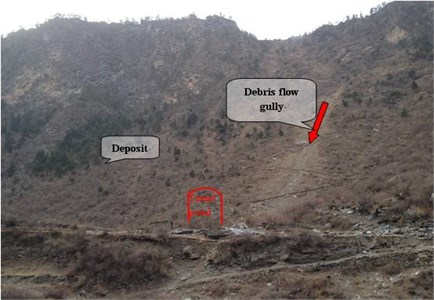### 4.1. Calculation for attribute measures of single index and comprehensive indices

Integrated degree of rock mass is taken as broken with its intactness index ${K}_{V}=$ 0.22; Lithological feature is soft for the saturated uniaxial compressive strength of the in-site rock sample ${R}_{c}=$5.47 MPa. For geological structural feature and weathering degree, the average joint spacing ${J}_{d}$ is 0.18 m by in-site measurement while the surrounding rock is completely weathered. Seismic intensity is identified as 8 according to the historical geological structure analysis. The average gradient of the slop at the tunnel exit area is 57°.

Fig. 2Diagram of tunnel geography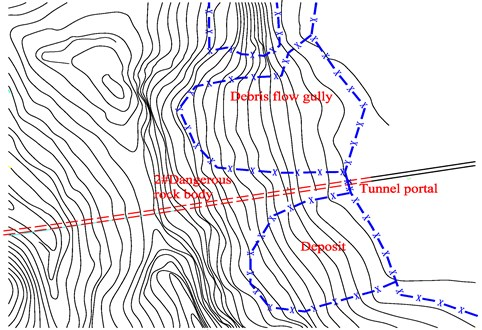Based on the specimen data (see Table 8) and the attribute measure functions of single index (see Table 7 in Appendix), the attribute measures of single index can be calculated. The comprehensive attribute measures of specimen can be calculated by Eq. (9), as shown in Table 8.

Table 8Calculation table of attribute measures

 Indices Measured value Attribute measures ${C}_{1}$ ${C}_{2}$ ${C}_{3}$ ${C}_{4}$ ${C}_{5}$ ${I}_{1}$ 0.22 0 0 0 0.3 0 ${I}_{2}$ 5.47 MPa 0 0 0.59 0.41 0 ${I}_{3}$ 0.18 m 0 0. 0.3 0.7 0 ${I}_{4}$ 0.09 0 0 0 0.4 0.6 ${I}_{5}$ 8 0 0 0 0.5 0.5 ${I}_{6}$ 57° 0 0 0 0.68 0.32 ${I}_{7}$ 720 mm 0 0.43 0.57 0 0 ${I}_{8}$ Excellent 1 0 0 0 0 Comprehensive attribute measures 0.090 0.040 0.177 0.396 0.147

### 4.2. Analysis of attribute recognition

The order of assessment set (${C}_{1}$, ${C}_{2}$, ${C}_{3}$, ${C}_{4}$, ${C}_{5}$) is taken as ${C}_{1}>{C}_{2}>{C}_{3}>{C}_{4}>{C}_{5}$ . When the value of $\lambda$ is adopted as 0.70, Eq. (11) can be written as the following form:

14
${k}_{0}=\mathrm{m}\mathrm{i}\mathrm{n}\left\{k:\sum _{l=1}^{k}{\mu }_{xl}\ge 0.70,1\le k\le 5\right\}.$

The stability grade of slope at Jiefangcun Tunnel portal is ${C}_{4}$ provided with rather high risk. The results derived from the extension assessment method  are consistent with the results in this paper. The rationality and feasibility of application of attribute mathematics theory in the risk assessment for slope stability at tunnel portal is verified.

The method used in this paper can solve the deviation of the geological qualitative analysis caused by human subjectivity. The influence of human subjectivity on selection of risk factors and level division is reduced to some extent based on the standard of hydrogeology and engineering geology and the experience of exports consultation. This method of risk evaluation of slope instability, considering the impact of excavation tunnel portal, could be used to make scheme comparison of tunnel portal construction and slope protection in the investigation stage; in the construction stage, appropriate risk treatment programs or measures could also be developed in accordance with the evaluation results to achieve the purpose of disaster prevention and mitigation, early warning and safe construction.

### 4.3. Tracking and treatments for construction risk of tunnel portal

To make risk solutions and enhance monitoring for avoiding or reducing loses, the risk assessment was carried out in practice. The tracking and treatment in tunneling process of Jiefangcun Tunnel were as follows:

The cracks were produced at many places at the surface, slope and primary supporting of tunnel with small-scale collapse after the portal excavation of Jiefangcun Tunnel (see Fig. 3). After the risk analysis and assessment, the following treatments were adopted to guarantee the construction safety: (1) the grouting reinforcement towards surrounding rock of tunnel portal was used; (2) the advanced grouting with small pipe was added to reinforce the forward surrounding rock; (3) the surface of tunnel ceiling was covered with striped cloth, and the net-suspended spraying was conducted to close the cracks; (4) the bias open cut tunnel was built; and (5) the temporary invert was added.

Fig. 3Tracking for construction risk of tunnel portal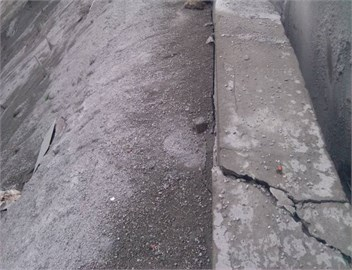a)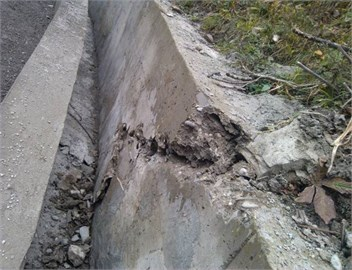b)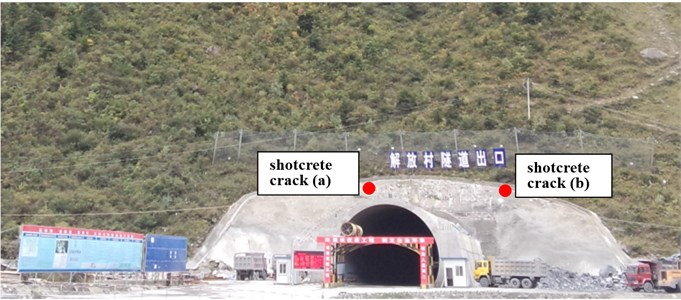c)

## 5. Conclusions

In the present paper, to ensure the construction safety of tunnel, the attribute recognition model of risk assessment for the slope stability at tunnel portal was established by using attribute mathematics theory. The integrated degree of rock mass, lithological feature, geological structural feature, weathering degree, seismic intensity, gradient, rainfall and construction factors were selected as the indices of the risk assessment for the stability slope at tunnel portal with grading criteria of each index. Moreover, the weights of the assessment indices were determined by the judgment matrix constructed from analytic hierarchy process. The attribute measurement functions were used to compute attribute measurement of single index and synthetic attribute measurement. The identification and classification of risk assessment for the slope stability at tunnel portal were assessed using the confidence criterion. For practical purposes, the stability of slope located in portal of an actual Tunnel, i.e., Jiefangcun Tunnel in Cheng-Lan Railway, was evaluated based on the previously established attribute recognition model. The corresponding risk treatment measures were then proposed based on the assessment results and monitor results to ensure the construction safety of tunnel portal.

#### Cited by

Environmental Risk Source Analysis and Classification of Zones: Subway Construction
2022 International Conference on Cloud Computing, Big Data and Internet of Things (3CBIT)
Collapse risk assessment of deep-buried tunnel during construction and its application
Risk assessment of highway structures in natural disaster for the property insurance
Study on slope stability due to the influence of excavation of the high-speed rail tunnel

23 October 2016
Accepted
04 May 2017
Published
30 June 2017
SUBJECTS
Seismic engineering and applications
Keywords
tunnel portal
slope stability
risk assessment
attribute recognition
Acknowledgements

This work was supported by the National Natural Science Foundation of China (51609138). Great appreciation goes to the editorial board and the reviewers of this paper.